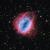# Knoblauch's blog## Octahedron

Octahedron is the third platonic solid. It consists of 8 faces (each is an equilateral triangle) and 6 vertices (each at the meeting of 4 faces).## Tetrahedron

The tetrahedron has the smallest number of faces in the five Platonic Solids, having only 4 faces. And in fact, four faces are the minimium requirement for a polyhedron. Other features of tetrahedron includes:

• Each face is an equilaterial triangle
• Each vertex is the meeting of 3 faces# Introduction

Platonic solids are polyhedron which satisfy 3 conditions

1. all its faces are congruent convex regular polygons,
2. none of its faces intersect except at their edges, and
3. the same number of faces meet at each of its vertices

## Hexahedron

The most commonly recognised platonic solid, also known as a cube.## An attempt at using recursion

All of my projects to date have required step by step instructions (some upwards of 300 lines of code!), so I decided to have a go at working out how to create a recursive code. After studying "Cayley graph 3D" working out in my head how the code was constructed, I was finally able to start my own. The following is based on an image of

Cayley Graph of the Free Product Z3 * Z5

After much trial and error I was able to develop ...## Snub dodecahedron

The Snub dodecahedron has 92 faces (12 pentagons, 80 equilateral triangles), 150 edges,  and 60 vertices.